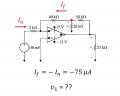# Operational Amplifer with negative feedack

#### Mohamed Mokhtar

Joined Sep 30, 2015
12
hi, my question is about an op-amp, i've already calculated the current passing through the 2 KΩ resistor which is the same current passing through the feedback branch, the problem is about getting the voltage across the 20 KΩ resistor , i just can't determine how to treat this resister ?? can i tell that the 20 KΩ resistor is in parallel with the 40 KΩ resitor since both of them are connected by two nodes (the first node is red node in the image and the second node is the ground since the 40 KΩ resistor has one node at 0V) ?#### crutschow

Joined Mar 14, 2008
32,932
Yes, for the purposes of calculating the voltage across the 20kΩ resistor you can treat it and the 40kΩ resistor as being in parallel since their other ends are both at ground potential (one real and one virtual).

But you do realize that the voltage at the node of the three resistors is independent of where the other end of the 20kΩ resistor is connected and is also independent of the value of the 20kΩ and 50kΩ resistors (as long as the op amp stays within its linear region). (?)

#### Mohamed Mokhtar

Joined Sep 30, 2015
12
Yes, for the purposes of calculating the voltage across the 20kΩ resistor you can treat it and the 40kΩ resistor as being in parallel since their other ends are both at ground potential (one real and one virtual).

But you do realize that the voltage at the node of the three resistors is independent of where the other end of the 20kΩ resistor is connected and is also independent of the value of the 20kΩ and 50kΩ resistors (as long as the op amp stays within its linear region). (?)
sorry i didn't get it , you mean that the voltage of the red node will never change till i cahnge the value of the 40 KΩ resistor ? why ?

#### crutschow

Joined Mar 14, 2008
32,932
sorry i didn't get it , you mean that the voltage of the red node will never change till i cahnge the value of the 40 KΩ resistor ? why ?
Well, as a student, that's something you should answer.
If you understand how the summing node of the op amp works then you will see that, for a given input, the red node voltage is only dependent upon the value of the 40kΩ resistor.
You already stated that If = In. So from that, Ohm's law tells you the answer.